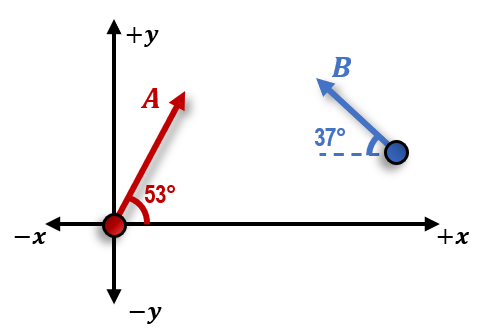## Physics

Learn the toughest concepts covered in Physics with step-by-step video tutorials and practice problems by world-class tutors

11. Momentum & Impulse

# Intro to Conservation of Momentum

1
concept

## Total Momentum of a System of Objects3m
Play a video:
2
Problem

Object A moves at 10 m/s at 53° and Object B moves at 5 m/s at –37° as shown below. Calculate the magnitude of the system’s total momentum if both objects have a mass of 2kg.3
concept

## Conservation Of Momentum5m
Play a video: